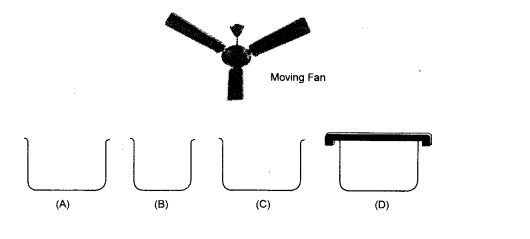# Look at the figure and suggest in`
Question:

Look at the figure and suggest in which of the vessels $A, B, C$ or $D$, the rate of evaporation will be the highest ? Explain.Solution:

Vessel $B$ has the smallest size while vessel $D$ is covered. Though vessel $A$ and $C$ are of same size, moving fan will accelerate evaporation. Therefore, the rate of evaporation will be highest in vessel $C$.